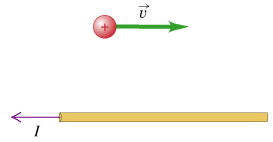# Problem: A long straight horizontal wire carries a current = 2.10 A to the left. A positive 1.00 C charge moves to the right at a distance 1.50 m above the wire at constant speed = 2000 m/s . (Figure 1) A. What are the magnitude and the direction of the magnetic force on the charge?B. Select the source(s) responsible for the magnetic field that exerts a force on the moving charge?a) the chargeb) the current-carrying wirec) the charge and the current-carrying wire

###### FREE Expert Solution

A. The magnitude of magnetic force:

$\overline{){{\mathbf{F}}}_{{\mathbf{B}}}{\mathbf{=}}{\mathbf{q}}{\mathbf{v}}{\mathbf{B}}}$

The magnitude of magnetic field:

$\overline{){\mathbf{B}}{\mathbf{=}}\frac{{\mathbf{\mu }}_{\mathbf{0}}\mathbf{I}}{\mathbf{2}\mathbf{\pi }\mathbf{r}}}$

Substituting:

89% (464 ratings)###### Problem DetailsA long straight horizontal wire carries a current = 2.10 A to the left. A positive 1.00 C charge moves to the right at a distance 1.50 m above the wire at constant speed = 2000 m/s . (Figure 1)

A. What are the magnitude and the direction of the magnetic force on the charge?

B. Select the source(s) responsible for the magnetic field that exerts a force on the moving charge?

a) the charge

b) the current-carrying wire

c) the charge and the current-carrying wire